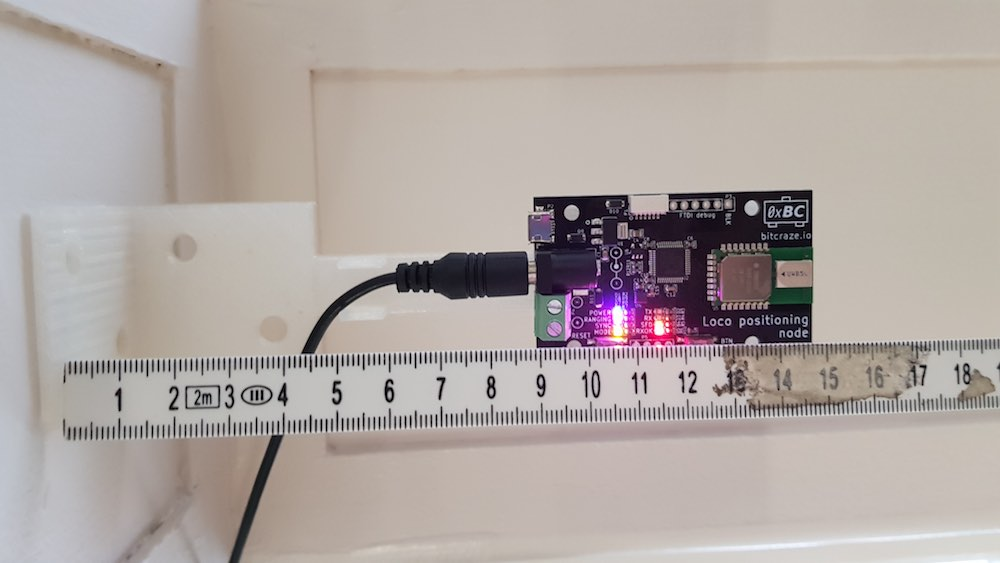# Automated anchor position estimation

One of the pain points when setting up the Loco Positioning system is to measure the anchor positions and enter them into the system. I wanted to see if I could automate this task and let the system calculate the positions, and if so understand what kind of precision to expect. I have spent a few Fun Fridays playing with this problem and this is what I have found so far.

The problem can be broken down into two parts:
1. How to calculate the anchor positions. What data is required?
2. How to define the coordinate system. To make it useful the user must to be able to define the coordinate system in a simple way.### How to calculate the anchor positions

The general idea of how to calculate the anchor positions is to set up a system of equations describing the distances between the anchors and/or the Crayzflie and solve for the anchor positions. The equations will be non linear and the (possibly naive) plan is to use the Gauss Newton method to solve the system.

To understand how to calculate the anchor positions we must first take a look at the data that is available. The Loco Positioning system can be run in two different modes: Two Way ranging (default mode) and TDoA.

#### Two way ranging

In the Two Way ranging mode we measure the distance between each anchor and the Crazyflie and to get enough data we must record ranging data for multiple positions. The anchor positions are unknown, and for each new Crazyflie position we add yet a new unknown position, on the other hand we measure the ranges to the anchors so these are knowns.

The equations used are simply to calculate the distance between the assumed position of each anchor and the Crazyflie and then subtracting it from the measured distance.

#### TDoA

In TDoA we measure the Time Difference of Arrival, that is the difference in distance to two anchors from the Crazyflie’s position. It is probably possible to use this information, but I was looking for a different solution here. In our new TDoA implementation that we have been playing with a bit, we get the distance between all anchors (calculated in the anchors) as a side effect.

In this case the Crazflie is not really needed and the equations describe the distance between assumed anchor positions versus measured distances.

### How to define the coordinate system

To get a useable positioning system, the coordinate system must be well defined and oriented in a practical direction. For example when writing a script you probably want (0, 0, 0) to be at some specific spot, the X-axis pointing in a certain direction, the Z-axis to point up and so on. My initial idea was to use the anchors to define the coordinate system, use anchor 0 as (0, 0, 0), let the X-axis pass through anchor 1 and so on. Just by looking at our flight lab I realised that this would be too limiting and decided that the coordinate system should be completely disconnected from the anchor positions, but still easy to define. I also realised that a really good way to tell the system about the desired coordinate system would be to move the Crazyflie around in space to show what you want. The solution is to place the Crazyflie at certain positions and click a button to record data at these positions. The steps I have chosen are:

1. Place the Crazyflie at (0, 0, 0)
2. Place the Crazyflie on the X-axis, X > 0
3. Place the Crazyflie in the XY-plane, Y > 0
4. Move the Crazyflie around in the space with continuous recording of data

In this scheme the XY-plane is typically the floor.

### Results

I have written basic implementations for both the Two Way ranging and TDoA modes and they seem to work reasonably well in simulations. I have also tested the Two Way ranging algorithm in our flight lab with mixed results. The solution converged in most cases but not always. When converging the estimated anchor positions ended up in the right region but some were off by up to a meter. Finally I did run the algorithm and fed the result into the system and managed to fly using the estimated positions which I find encouraging.

I will continue to work on this as a Friday Fun project and maybe it will make its way into the client code base at some point in the future? There are probably better ways to estimate the anchor positions and more clever algorithms, feel free to share them in the comments.

## 4 comments on “Automated anchor position estimation”

•Lionel says:

Hi, I’m aware of two other methods to estimate the anchor positions :
– the first one uses the multidimensional scaling algorithm (MDS) and works by measuring the distance between each anchors and possibly the crazyflie. The algorithm will output the relative position between the anchors.
– the second method is simultaneous localization and mapping (SLAM) which estimate the anchor positions in addition to the crazyflie pose. The algorithm would use the crazyflie or IMU model for the prediction and the range measurements from the anchors for the correction step.

•Kristoffer Richardsson says:

Thanks for the input!

•ydx says:

Hello!
In the tutorials of getting started with the LPS,when I Click the button “Get from anchors”, i can’t get the positions with the values currently stored in the anchors,what’s the reason?
thank you.

•Kimberly McGuire says: